# RS Aggarwal Solutions for Class 6 Chapter 2 Factors and Multiples Exercise 2D

RS Aggarwal Solutions for Class 6 Chapter 2 Factors and Multiples Exercise 2D PDF are provided here. The exercise wise solutions designed by faculty at BYJU’S help students in solving RS Aggarwal textbook problems. The solutions are in explanatory manner to improve conceptual knowledge among the students. The primary objective of preparing the solutions is to make the subject easier for students.

## Download PDF of RS Aggarwal Solutions for Class 6 Chapter 2 Factors and Multiples Exercise 2D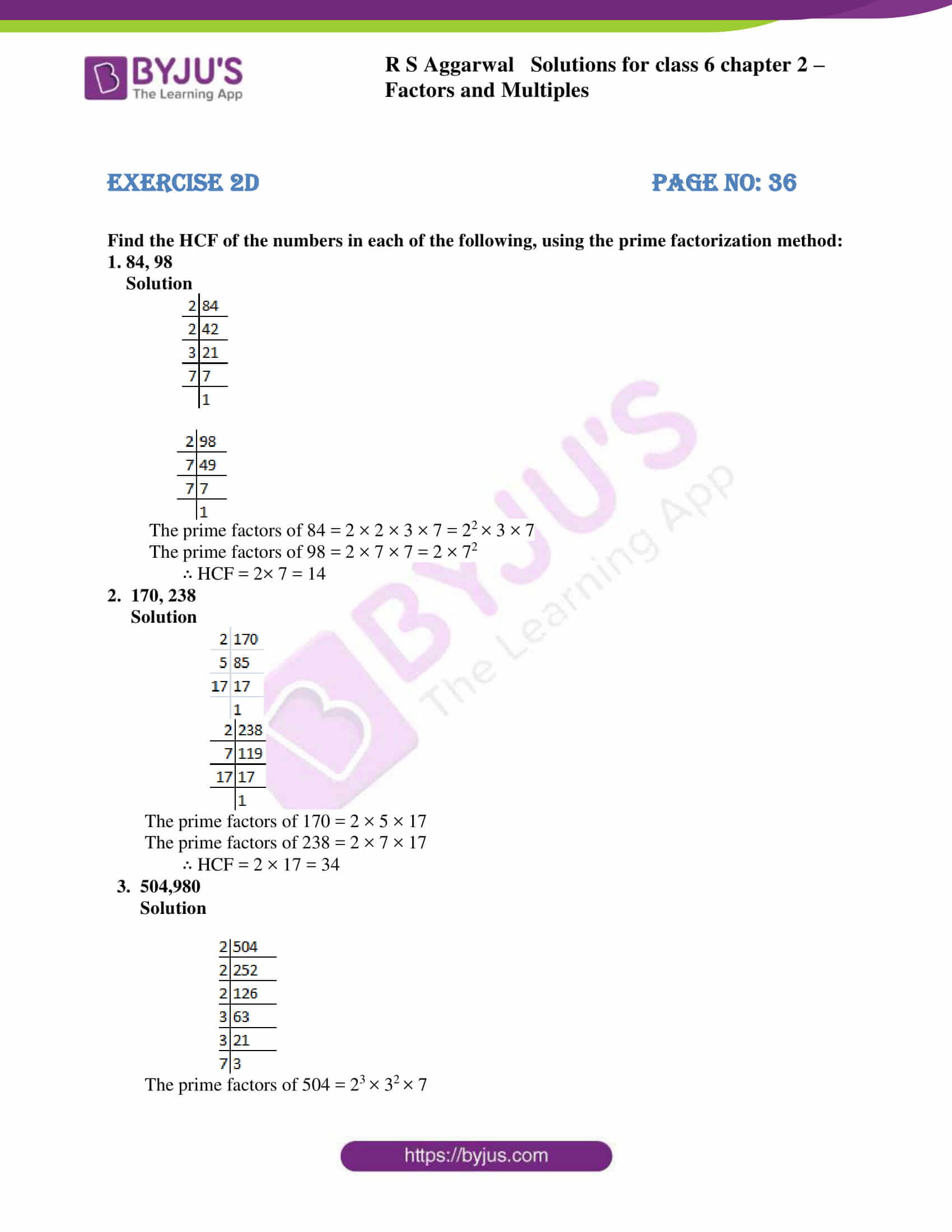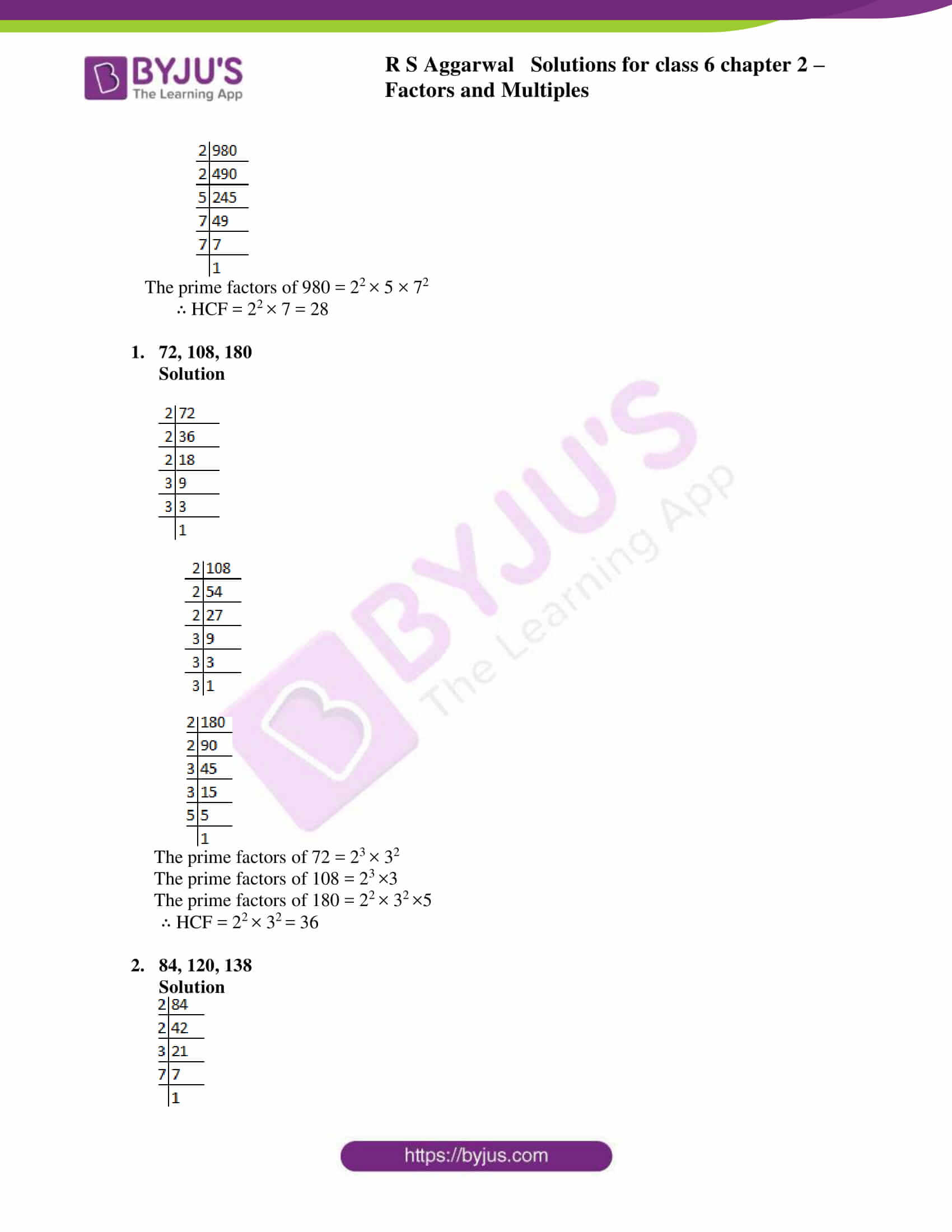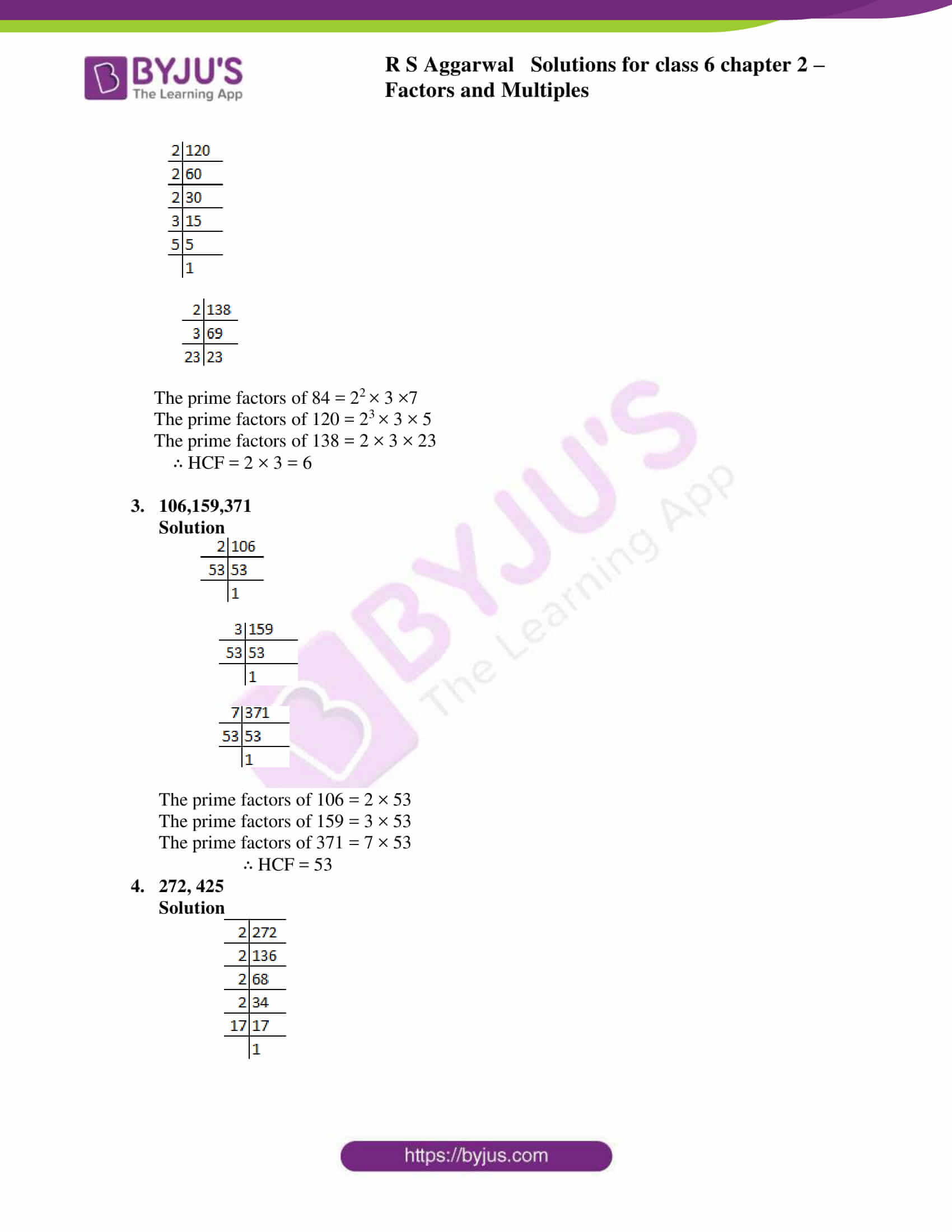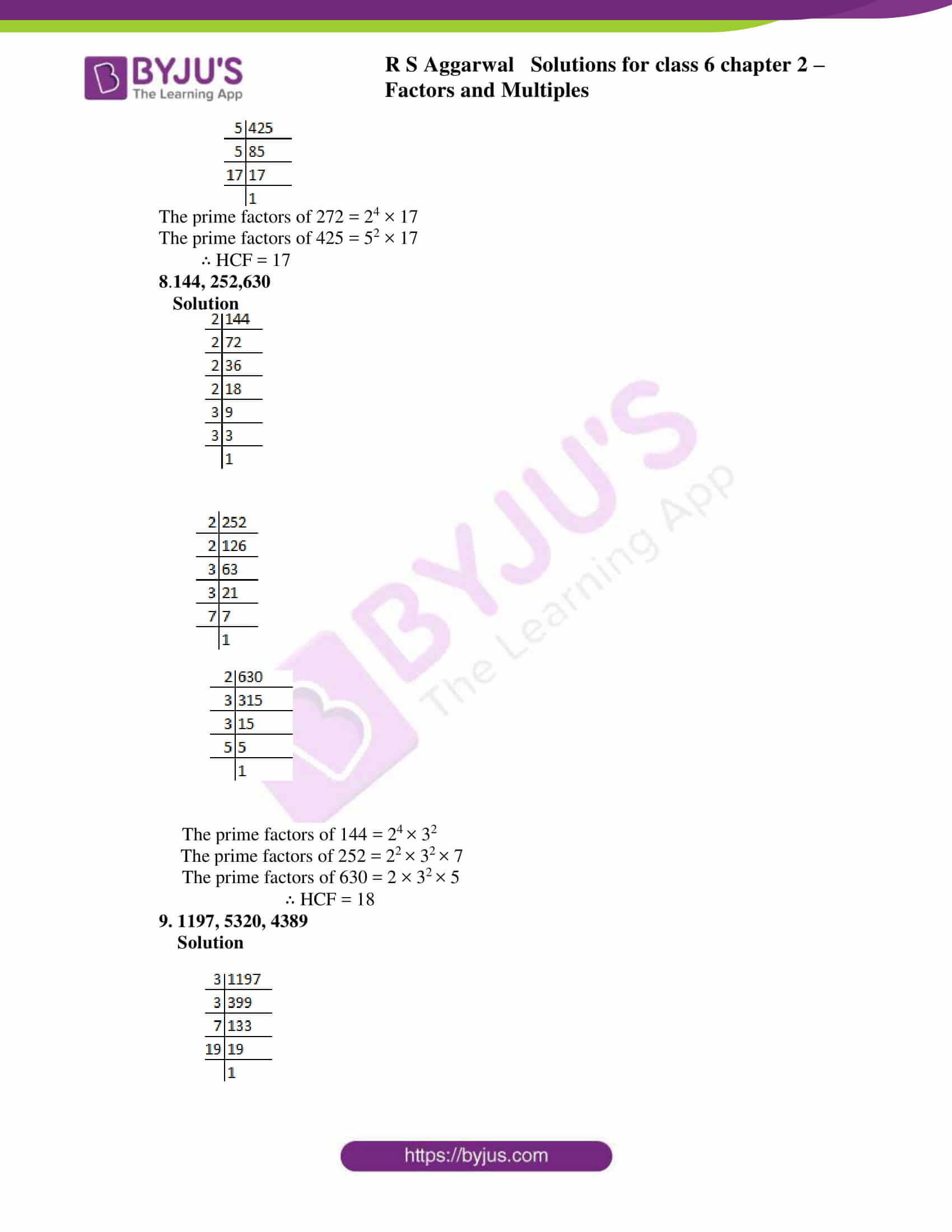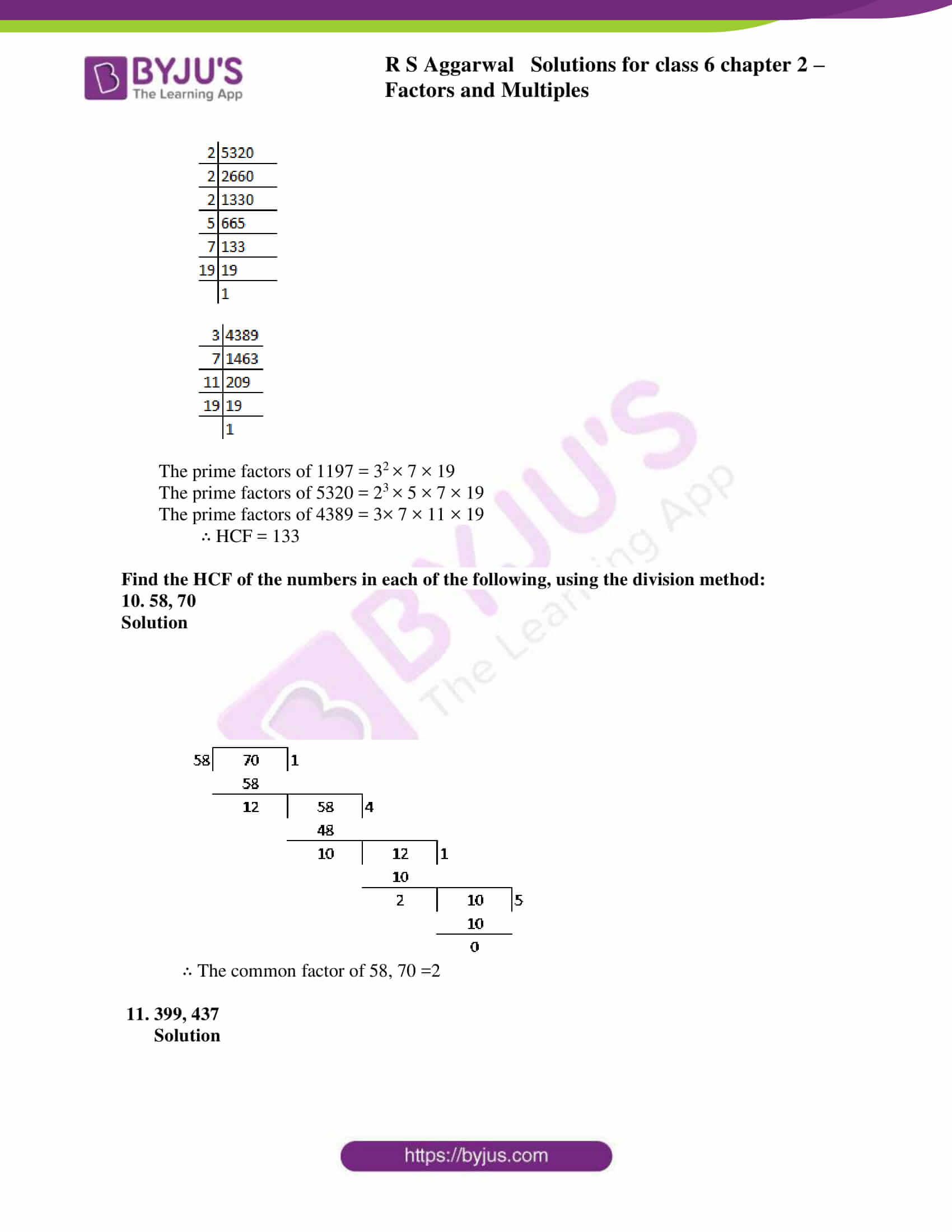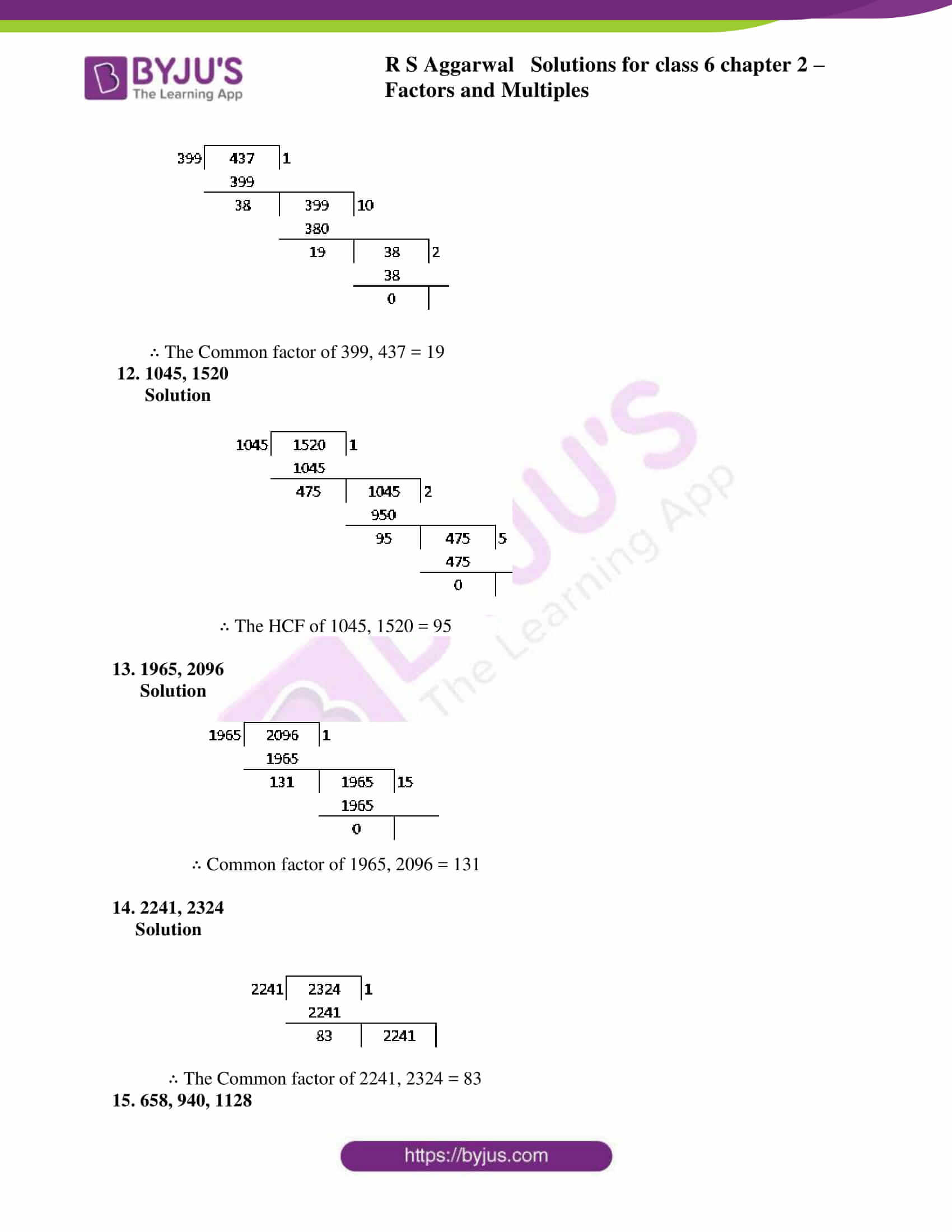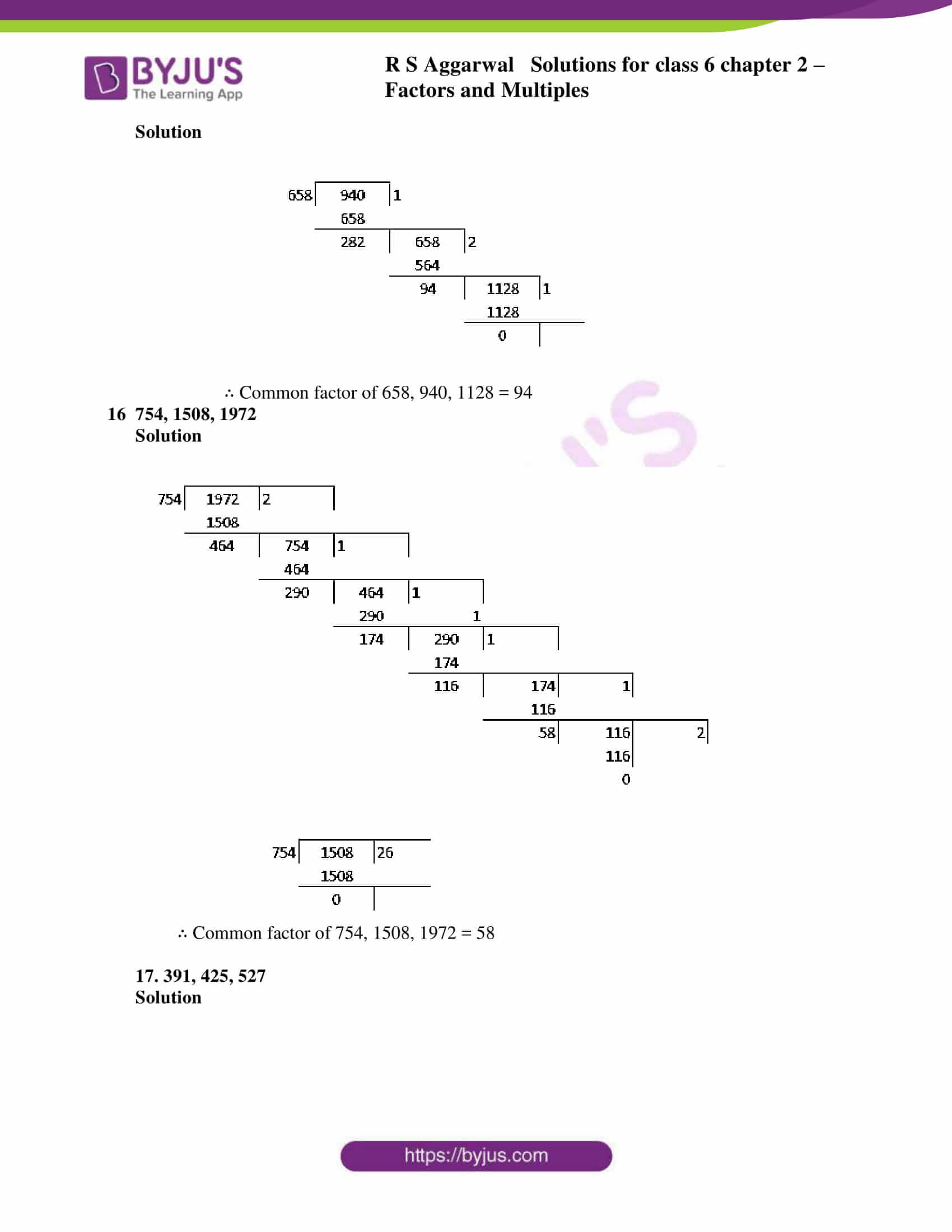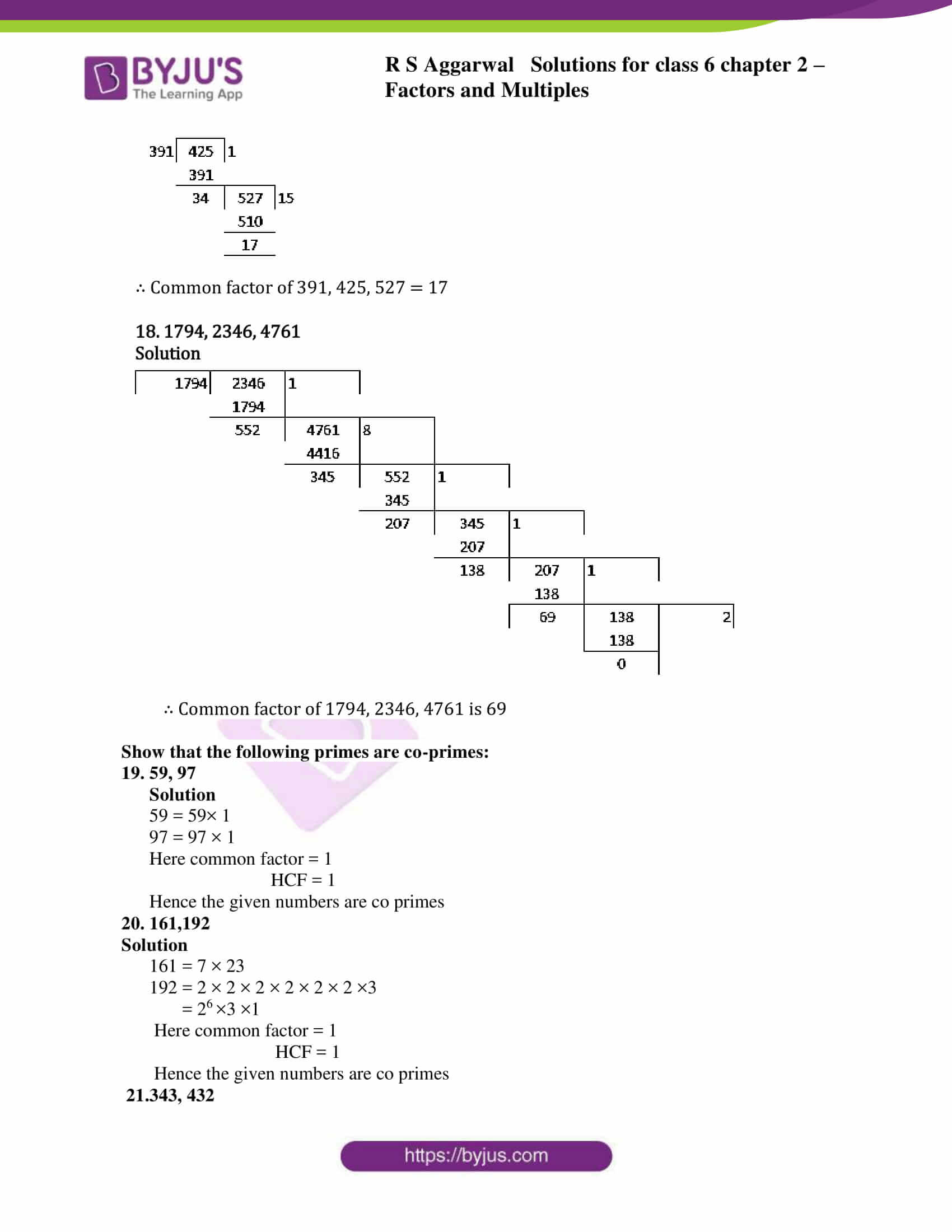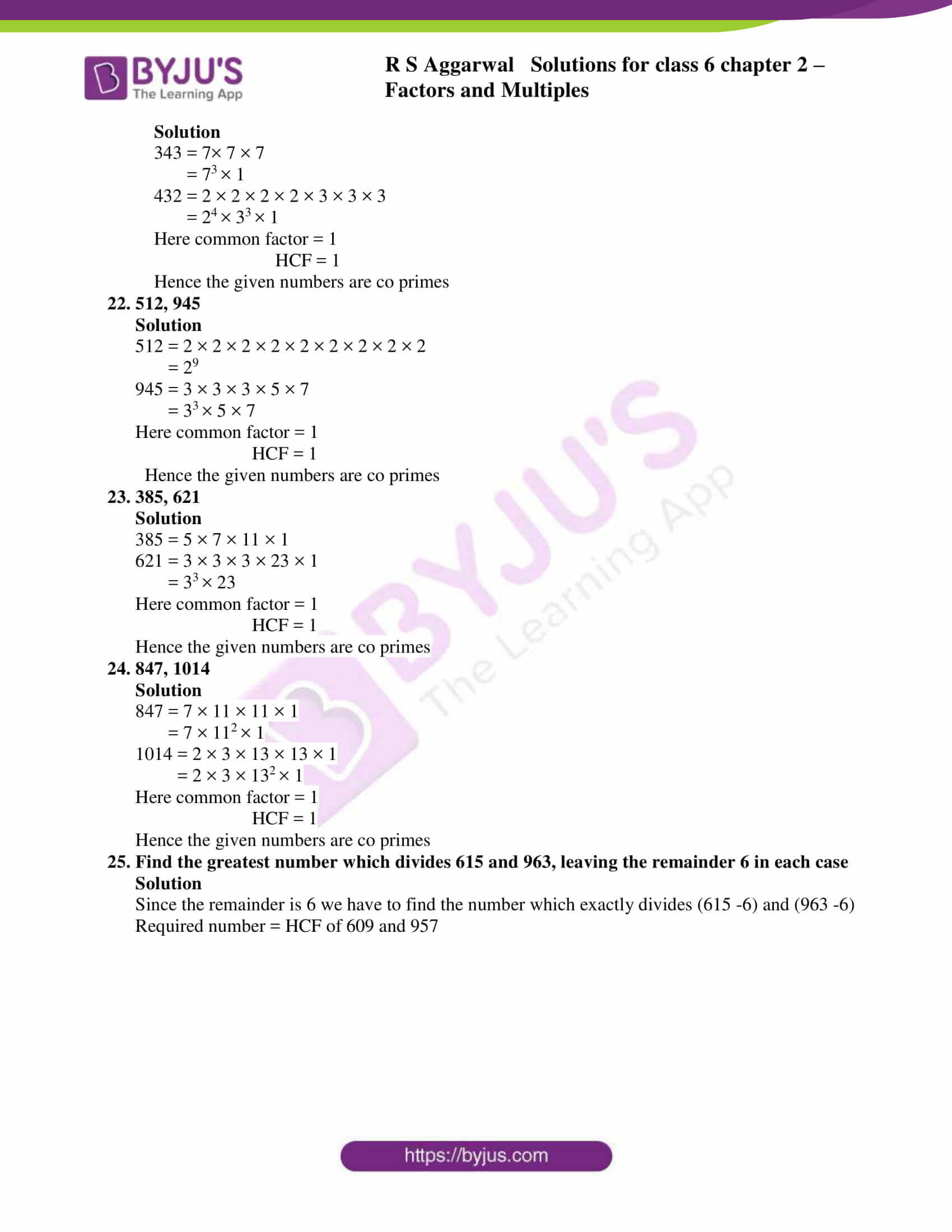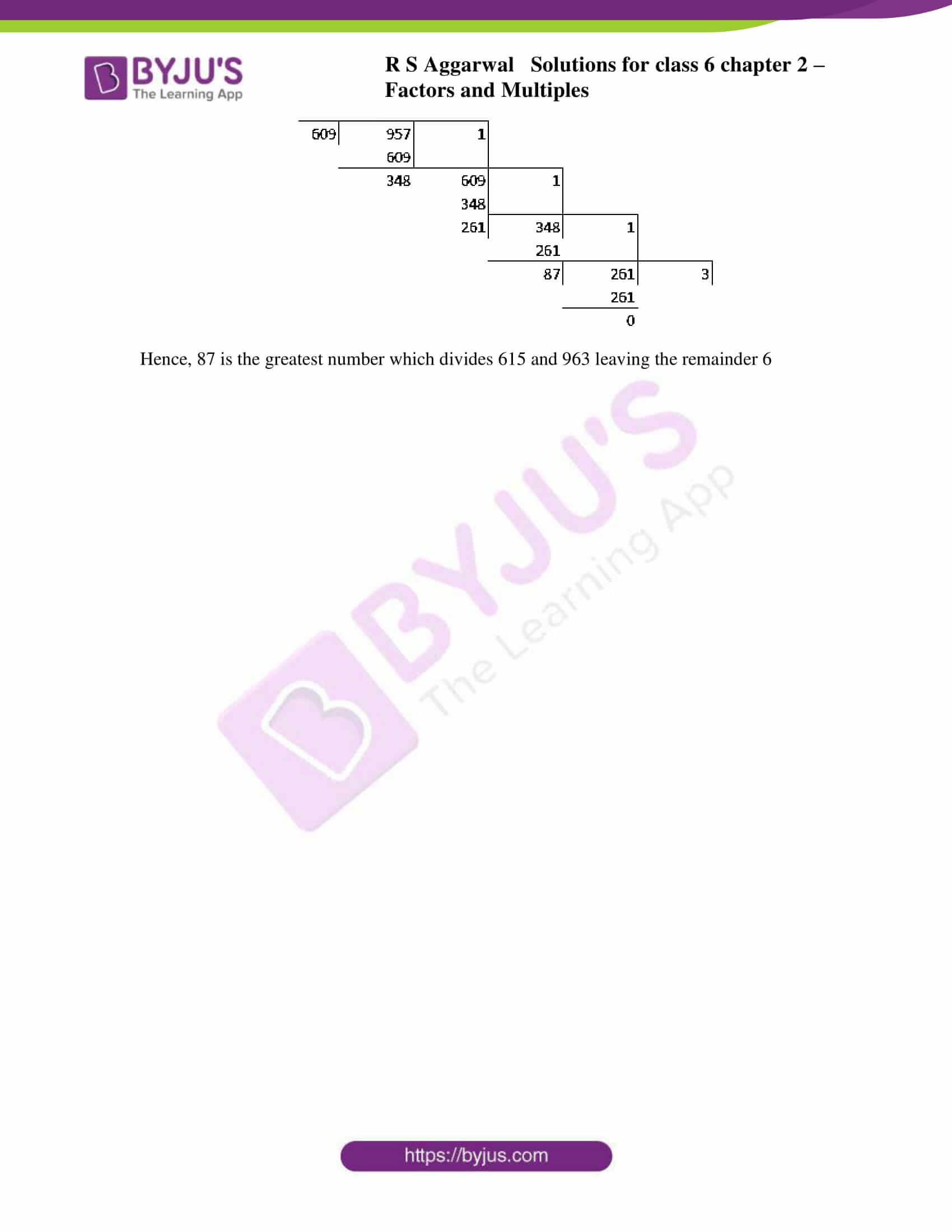### Access answers to RS Aggarwal Solutions for Class 6 Chapter 2 Factors and Multiples Exercise 2D

Find the HCF of the numbers in each of the following, using the prime factorization method:

1. 84, 98

Solution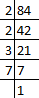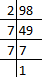The prime factors of 84 = 2 × 2 × 3 × 7 = 22 × 3 × 7

The prime factors of 98 = 2 × 7 × 7 = 2 × 72

∴ HCF = 2× 7 = 14

2. 170, 238

Solution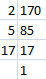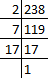The prime factors of 170 = 2 × 5 × 17

The prime factors of 238 = 2 × 7 × 17

∴ HCF = 2 × 17 = 34

3. 504,980

Solution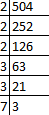The prime factors of 504 = 23 × 32 × 7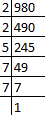The prime factors of 980 = 22 × 5 × 72

∴ HCF = 22 × 7 = 28

1. 72, 108, 180

Solution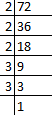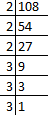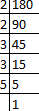The prime factors of 72 = 23 × 32

The prime factors of 108 = 23 ×3

The prime factors of 180 = 22 × 32 ×5

∴ HCF = 22 × 32 = 36

1. 84, 120, 138

Solution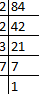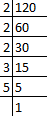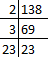The prime factors of 84 = 22 × 3 ×7

The prime factors of 120 = 23 × 3 × 5

The prime factors of 138 = 2 × 3 × 23

∴ HCF = 2 × 3 = 6

1. 106,159,371

Solution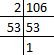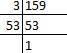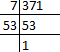The prime factors of 106 = 2 × 53

The prime factors of 159 = 3 × 53

The prime factors of 371 = 7 × 53

∴ HCF = 53

1. 272, 425

Solution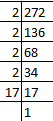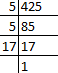The prime factors of 272 = 24 × 17

The prime factors of 425 = 52 × 17

∴ HCF = 17

8.144, 252,630

Solution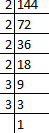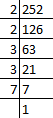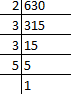The prime factors of 144 = 24 × 32

The prime factors of 252 = 22 × 32 × 7

The prime factors of 630 = 2 × 32 × 5

∴ HCF = 18

9. 1197, 5320, 4389

Solution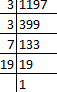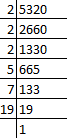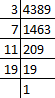The prime factors of 1197 = 32 × 7 × 19

The prime factors of 5320 = 23 × 5 × 7 × 19

The prime factors of 4389 = 3× 7 × 11 × 19

∴ HCF = 133

Find the HCF of the numbers in each of the following, using the division method:

10. 58, 70

Solution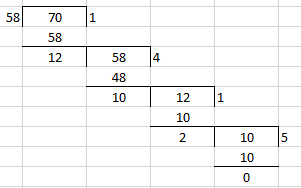∴ The common factor of 58, 70 =2

11. 399, 437

Solution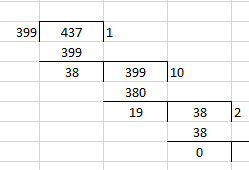∴ The Common factor of 399, 437 = 19

12. 1045, 1520

Solution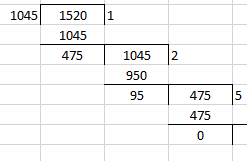∴ The HCF of 1045, 1520 = 95

13. 1965, 2096

Solution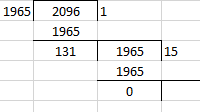∴ Common factor of 1965, 2096 = 131

14. 2241, 2324

Solution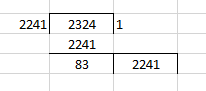∴ The Common factor of 2241, 2324 = 83

15. 658, 940, 1128

Solution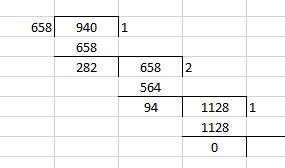∴ Common factor of 658, 940, 1128 = 94

1. 754, 1508, 1972

Solution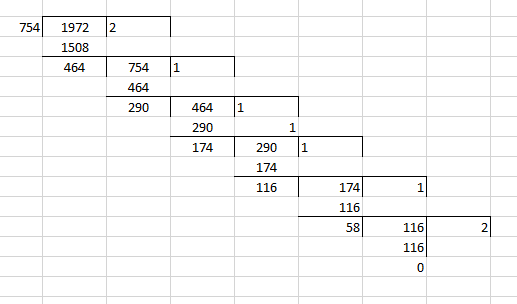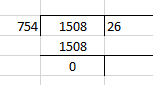∴ Common factor of 754, 1508, 1972 = 58

17. 391, 425, 527

Solution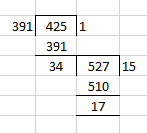∴ Common factor of 391, 425, 527 = 17

18. 1794, 2346, 4761

Solution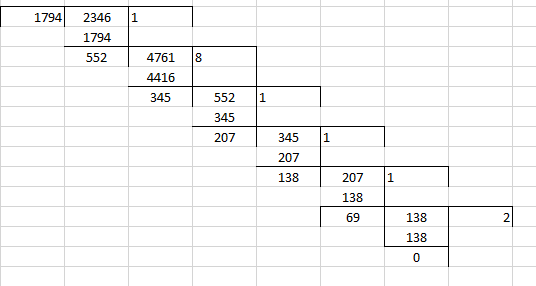∴ Common factor of 1794, 2346, 4761 is 69

Show that the following primes are co-primes:

19. 59, 97

Solution

59 = 59× 1

97 = 97 × 1

Here common factor = 1

HCF = 1

Hence the given numbers are co primes

20. 161,192

Solution

161 = 7 × 23

192 = 2 × 2 × 2 × 2 × 2 × 2 ×3

= 26 ×3 ×1

Here common factor = 1

HCF = 1

Hence the given numbers are co primes

21.343, 432

Solution

343 = 7× 7 × 7

= 73 × 1

432 = 2 × 2 × 2 × 2 × 3 × 3 × 3

= 24 × 33 × 1

Here common factor = 1

HCF = 1

Hence the given numbers are co primes

22. 512, 945

Solution

512 = 2 × 2 × 2 × 2 × 2 × 2 × 2 × 2 × 2

= 29

945 = 3 × 3 × 3 × 5 × 7

= 33 × 5 × 7

Here common factor = 1

HCF = 1

Hence the given numbers are co primes

23. 385, 621

Solution

385 = 5 × 7 × 11 × 1

621 = 3 × 3 × 3 × 23 × 1

= 33 × 23

Here common factor = 1

HCF = 1

Hence the given numbers are co primes

24. 847, 1014

Solution

847 = 7 × 11 × 11 × 1

= 7 × 112 × 1

1014 = 2 × 3 × 13 × 13 × 1

= 2 × 3 × 132 × 1

Here common factor = 1

HCF = 1

Hence the given numbers are co primes

25. Find the greatest number which divides 615 and 963, leaving the remainder 6 in each case

Solution

Since the remainder is 6 we have to find the number which exactly divides (615 -6) and (963 -6)

Required number = HCF of 609 and 957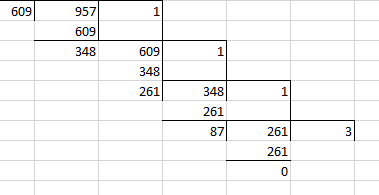Hence, 87 is the greatest number which divides 615 and 963 leaving the remainder 6

## RS Aggarwal Solutions for Class 6 Chapter 2 Factors and Multiples Exercise 2D

RS Aggarwal Solutions for Class 6 Chapter 2 Factors and Multiples Exercise 2D include the questions based on finding out the HCF and LCM using prime factorization and division method. Topics which are mentioned in other exercises are

• Finding prime and composite numbers from 1 to 100
• General properties of divisibility
• Prime factorization
• Properties of HCF and LCM of given numbers

Students can solve RS Aggarwal textbook using PDF of solutions to perform better in the exam. It helps students understand the other methods which can be used in solving the same problem in a shorter duration.